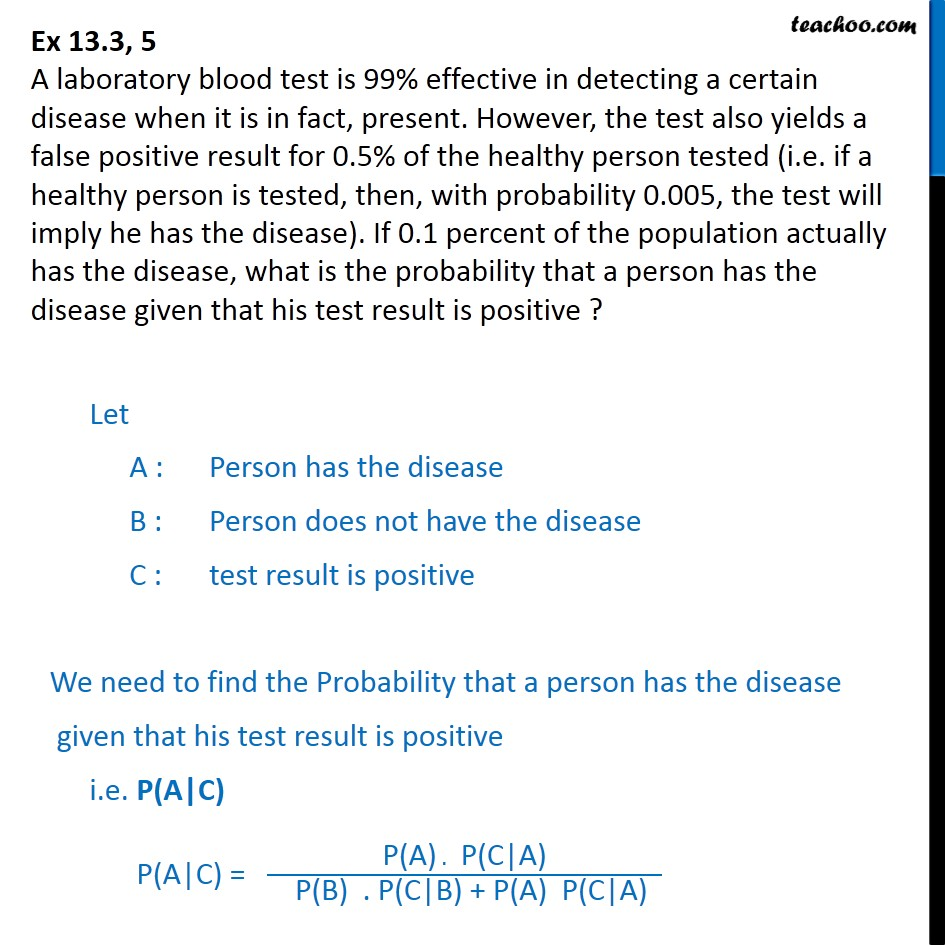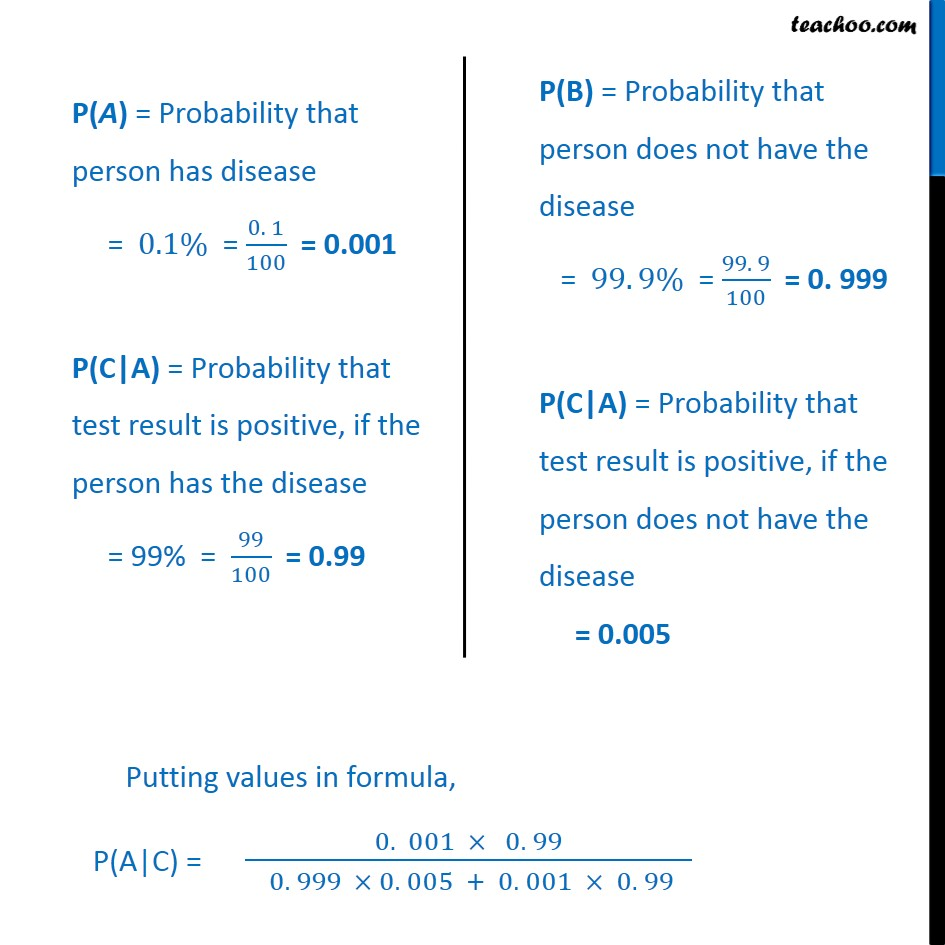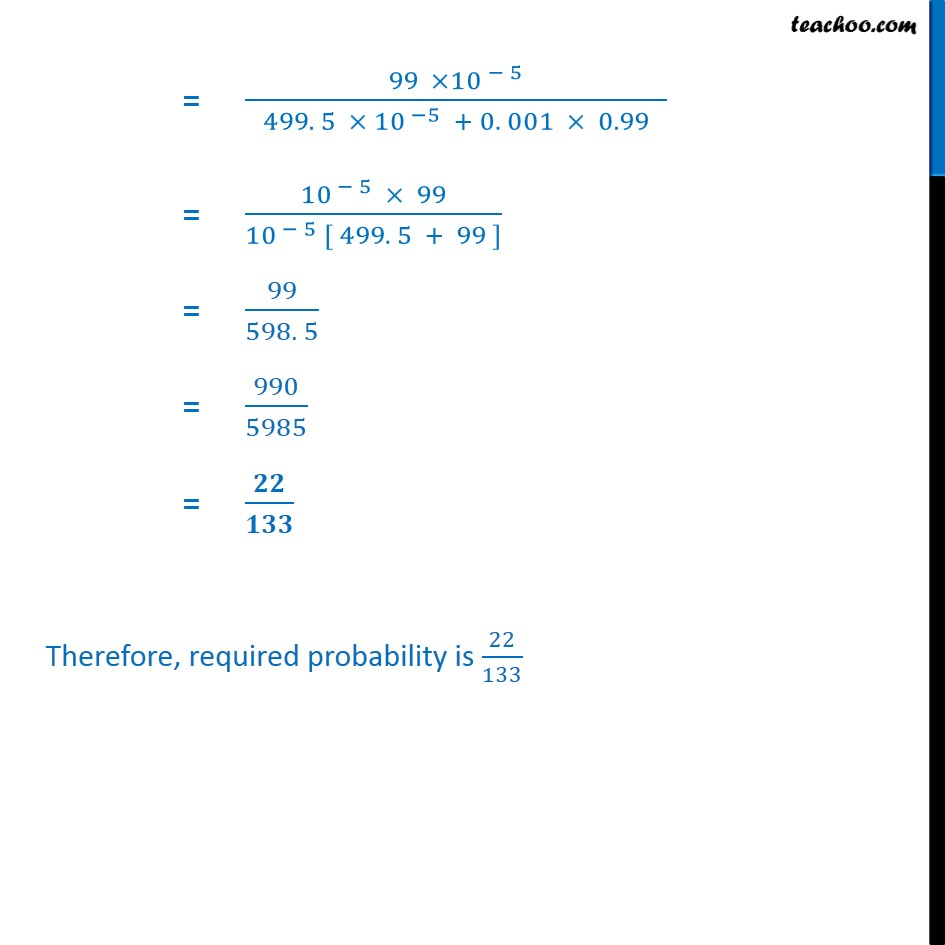Ex 13.3

Chapter 13 Class 12 Probability
Serial order wiseGet live Maths 1-on-1 Classs - Class 6 to 12

### Transcript

Ex 13.3, 5 A laboratory blood test is 99% effective in detecting a certain disease when it is in fact, present. However, the test also yields a false positive result for 0.5% of the healthy person tested (i.e. if a healthy person is tested, then, with probability 0.005, the test will imply he has the disease). If 0.1 percent of the population actually has the disease, what is the probability that a person has the disease given that his test result is positive ? Let A : Person has the disease B : Person does not have the disease C : test result is positive We need to find the Probability that a person has the disease given that his test result is positive i.e. P(A|C) P(A|C) = P(A) . P(C|A) P(B) . P(C|B) + P(A) P(C|A) Putting values in formula, P(A|C) = 0. 001 0. 99 0. 999 0. 005 + 0. 001 0. 99 = 99 10 5 499. 5 10 5 + 0. 001 0.99 = 10 5 99 10 5 [ 499. 5 + 99 ] = 99 598. 5 = 990 5985 = Therefore, required probability is 22 133